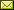## A Rigorous Extension of the Schönhage-Strassen Integer Multiplication Algorithm Using Complex Interval Arithmetic

 Thomas Steinke Raazesh Sainudiin

 Multiplication of n-digit integers by long multiplication requires O(n^2) operations and can be time-consuming. In 1970 A. Schoenhage and V. Strassen published an algorithm capable of performing the task with only O(n log(n)) arithmetic operations over the complex field C; naturally, finite-precision approximations to C are used and rounding errors need to be accounted for. Overall, using variable-precision fixed-point numbers, this results in an O(n(log(n))^(2+Epsilon))-time algorithm. However, to make this algorithm more efficient and practical we need to make use of hardware-based floating-point numbers. How do we deal with rounding errors? and how do we determine the limits of the fixed-precision hardware? Our solution is to use interval arithmetic to guarantee the correctness of results and determine the hardware's limits. We examine the feasibility of this approach and are able to report that 75,000-digit base-256 integers can be handled using double-precision containment sets. This clearly demonstrates that our approach has practical potential; however, at this stage, our implementation does not yet compete with commercial ones, but we are able to demonstrate the feasibility of this technique.

In Xizhong Zheng and Ning Zhong: Proceedings Seventh International Conference on Computability and Complexity in Analysis (CCA 2010), Zhenjiang, China, 21-25th June 2010, Electronic Proceedings in Theoretical Computer Science 24, pp. 151–159.
Published: 3rd June 2010.

 ArXived at: http://dx.doi.org/10.4204/EPTCS.24.19 bibtex PDF

 Comments and questions to:eptcs@eptcs.org For website issues:webmaster@eptcs.org